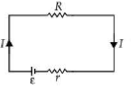Ohm's law
Question

# The internal resistance of a 2.1 V cell which gives a current of 0.2 A through a resistance of $10\Omega$ is

Easy
Solution

## $I=\frac{\epsilon }{R+r}or\text{\hspace{0.17em}\hspace{0.17em}}IR+Ir=\epsilon$Get Instant Solutions